By default, between two keys, the value of an animated parameter is updated in the form of a regular movement between the value given by the start key and the value given by the end key. A straight curve is obtained.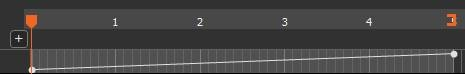You can modify the type of curve by right-clicking on it and selecting Change curve in the contextual menu.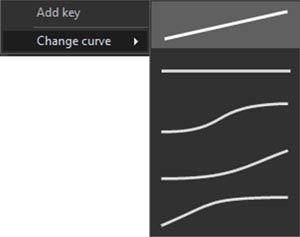There are five possible types of curves:

 Curve Name Effect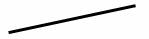Linear The parameter varies regularly from the start value to the end value.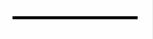Flat then jump The parameter keeps the starting value, then suddenly jumps to the end value. A discontinuity effect is achieved.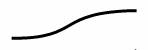Acceleration then deceleration The parameter is first modified slowly, then the modification speed accelerates, and finally decreases for a smooth arrival.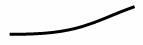Acceleration then linear The parameter is first changed slowly and then the change rate is accelerated to achieve a linear behavior.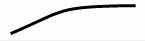Linear then deceleration A linear behavior is first achieved and then the speed of modification decreases for a smooth arrival.

Below is an example of a Flat then jump curve.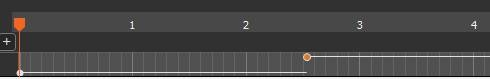The Flat then jump curve is very different from the others since it leads to brutal effects and discontinuities in the animation.

The difference between the other types of curve are mainly seen on parameters that have a significant impact on the terrain.

For parameters that have a less marked impact on the terrain, the difference between the different types of curve is more difficult to perceive.